Function Repository Resource:

# Involutions

List permutations comprised only of cycles of size two or less

Contributed by: Wolfram Staff (original content by Sriram V. Pemmaraju and Steven S. Skiena)
 ResourceFunction["Involutions"][l] gives the list of involutions of the elements in the list l. ResourceFunction["Involutions"][n] gives involutions for Range[n]. ResourceFunction["Involutions"][…,"Cycles"] gives involutions in their cycle representation.

## Details and Options

ResourceFunction["Involutions"] does not necessarily return the result in canonical order.
ResourceFunction["Involutions"][n,] is equivalent to ResourceFunction["Involutions"][Range[n],].

## Examples

### Basic Examples (3)

All involutive permutations of a list:

 In:=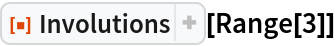Out=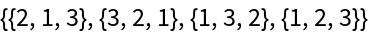Get the same result by specifying the size:

 In:=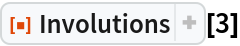Out=Cycle representations of the involutions:

 In:=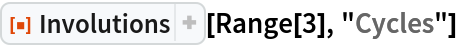Out=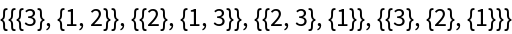In:=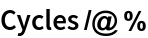Out=### Properties and Relations (4)

Permuting involutions with themselves gives the identity permutation:

 In:=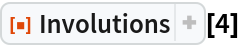Out=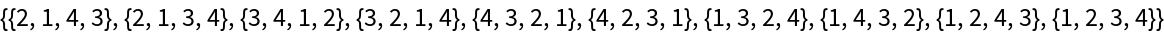In:=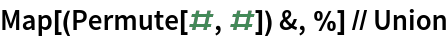Out=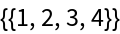The result given by Involutions can be verified using the resource function PermutationInvolutionQ:

 In:=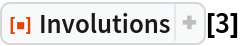Out=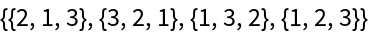In:=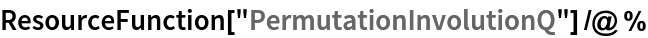Out=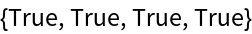Involutions can be obtained from a list of permutations:

 In:=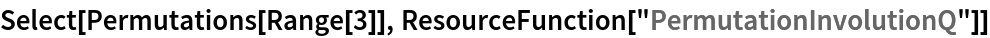Out=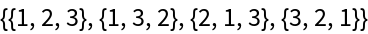In:=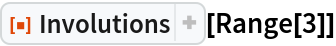Out=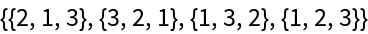In:=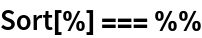Out=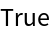The number of involutions is given by the resource function InvolutionCount:

 In:=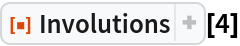Out=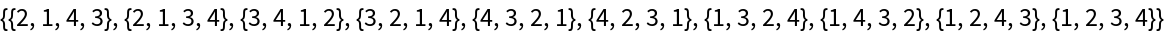In:=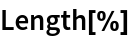Out=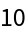In:=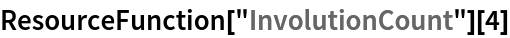Out=## Version History

• 1.0.0 – 26 June 2020